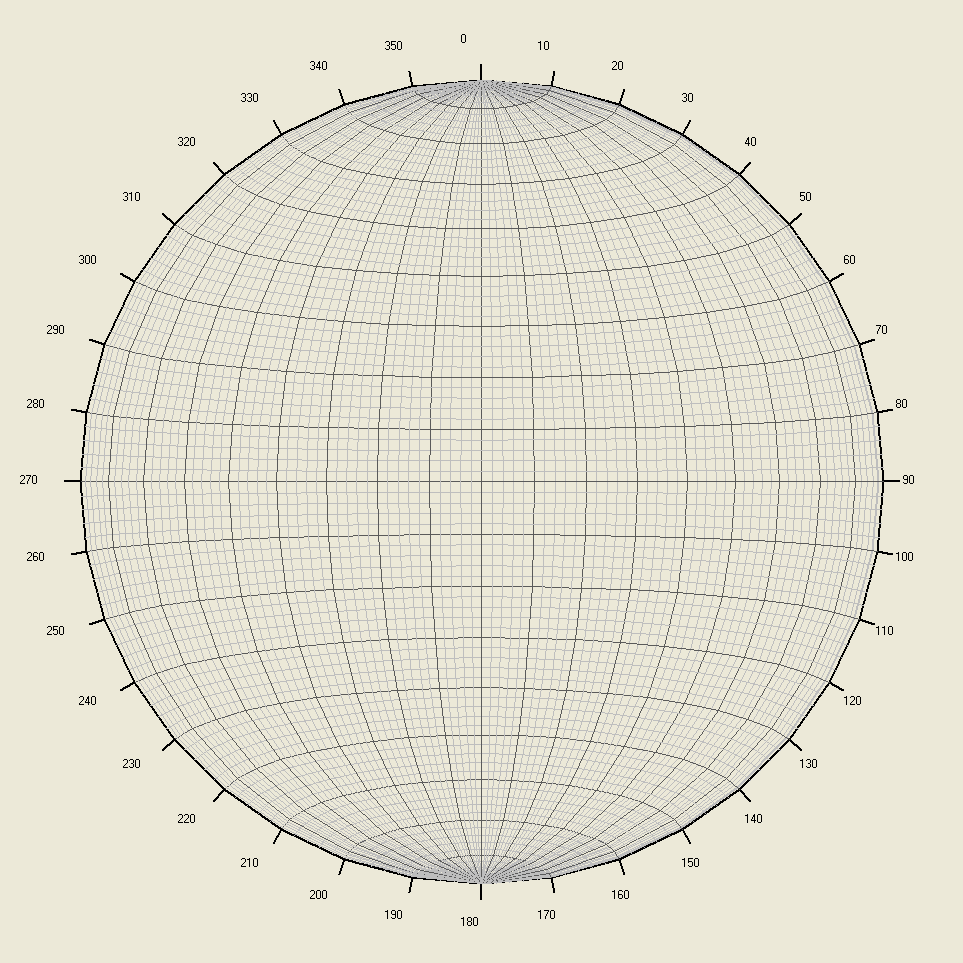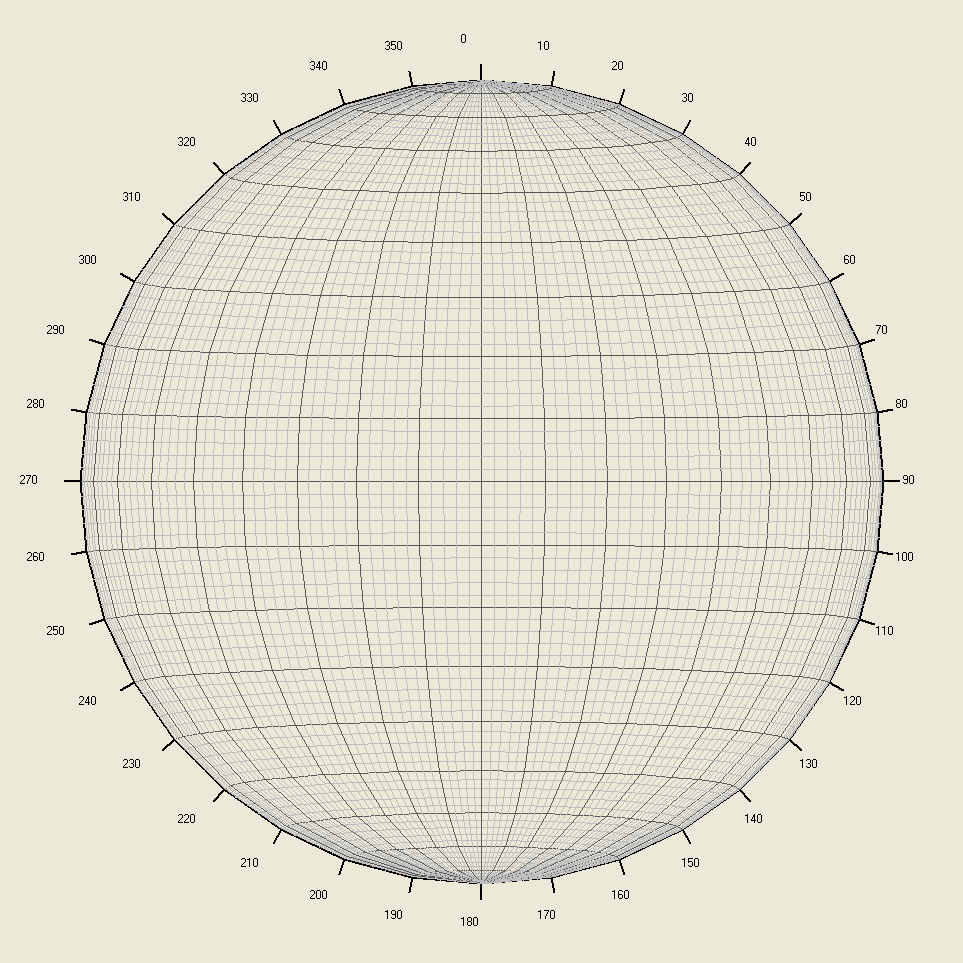## TU ANUNCIO / YOUR PUBLICITY

AQUÍ PODRÍA ESTAR TU ANUNCIO: / HERE COULD BE YOUR AD E-mail

## domingo, 26 de octubre de 2014

### Dip, direction, and the Schmidt's Stereographic projection / Buzamiento, rumbo y el diagrama de Schmidt

There are many good websites that tell us what it is. For example:
Hay muy buenas páginas de internet que nos dicen en qué consiste. Por ejemplo:

http://en.wikipedia.org/wiki/Lambert_azimuthal_equal-area_projection

http://en.wikipedia.org/wiki/Stereographic_projection

http://en.wikipedia.org/wiki/Schmidt_net#Construction

But ... seriously. How is it done? / Pero... en serio. ¿Cómo se hace?

Here I put a piece of software we use (en VisualBasic):
Aquí pongo un extracto del programa que utilizamos (in VB):
http://carreteras-laser-escaner.blogspot.com.es/

Picture1.Cls
Picture1.ScaleHeight = 12:Picture1.ScaleWidth = 12:Picture1.DrawWidth = 2
rr = 5: rr2 = 6
po = 2
For j = 0 To 350 Step 10: i = j * 0.017453278: i2 = i + 0.17453278:a2 = rr * Cos(i) + rr2: b2 = rr * Sin(i) + rr2: c2 = rr * Cos(i2) + rr2: d2 = rr * Sin(i2) + rr2:a1 = (rr + 0.2) * Cos(i) + rr2: b1 = (rr + 0.2) * Sin(i) + rr2:Picture1.Line (a2, b2)-(c2, d2): Picture1.Line (a2, b2)-(a1, b1)
b2 = -(rr + 0.5) * Cos(i) + rr2: a2 = (rr + 0.5) * Sin(i) + rr2:Picture1.CurrentX = a2 - 0.3: Picture1.CurrentY = b2 - 0.1: Picture1.Print j: Next j
For h = 2 To 178 Step 2: colore = RGB(160, 160, 160): If h Mod 10 = 0 Then colore = RGB(90, 90, 90) Else colore = RGB(190, 190, 190) :an = h * 0.017453278: cosa = Cos(an): sena = Sin(an): buz = 0: cosb = Cos(buz): sinb = Sin(buz):
For j = 0 To 170 Step 10: i = j * 0.017453278: i2 = j + 0.17453278: cosii = Cos(i): cosi2 = Cos(i2): sinii = Sin(i): sini2 = Sin(i2): x2 = rr * sinii * cosa: Z2 = -rr * sinii * sena: y2 = rr * cosii: xx = Z2 * x2 / (rr * po - Z2) + x2: yy = Z2 * y2 / (rr * po - Z2) + y2: x2 = xx::y2 = yy: x1 = rr * sini2 * cosa: Z1 = -rr * sini2 * sena: y1 = rr * cosi2
xx = Z1 * x1 / (rr * po - Z1) + x1: yy = Z1 * y1 / (rr * po - Z1) + y1
x1 = xx::y1 = yy: a1 = x2 * cosb + y2 * sinb: b1 = x2 * sinb - y2 * cosb: c1 = x1 * cosb + y1 * sinb: d1 = x1 * sinb - y1 * cosb: Picture1.Line (a1 + 6, b1 + 6)-(c1 + 6, d1 + 6), colore: Next j: Picture1.DrawWidth = 1
For j = -10 To -180 Step -10: i = j * 0.017453278: i2 = j + 0.17453278: y2 = rr * cosa:x2 = rr * sena * Cos(i):Z2 = rr * sena * Sin(i): xx = Z2 * x2 / (rr * po - Z2) + x2: yy = Z2 * y2 / (rr * po - Z2) + y2: x2 = xx + rr2: y2 = yy + rr2: y1 = rr * cosa:x1 = rr * sena * Cos(i2):Z1 = rr * sena * Sin(i2): xx = Z1 * x1 / (rr * po - Z1) + x1: yy = Z1 * y1 / (rr * po - Z1) + y1: x1 = xx + rr2: y1 = yy + rr2: Picture1.Line (x1, y1)-(x2, y2), colore

This makes the next image in Picture1:
Esto produce la siguiente imagen en Picture1:po=1 (view height: 1 radious / altura del punto de vista :1 radio)po=2 (view height: 2 radious / altura del punto de vista:2 radios)po=3 (view height: 3 radious / altura del punto de vista: 3 radios)po=4 (view height: 4 radious / altura del punto de vista: 4 radios)po=10 (view height: 10 radious / altura del punto de vista:10 radios)

## domingo, 19 de octubre de 2014

### Solution of Fermat's theorem on a margin / Solución del Teorema de Fermat en un margen

Although it is already solved, here I present my brief solution on "more than a margin"
Aunque ya está resuelto aquí expongo mi breve solución en "algo más que un margen"

#### Initial trick

A solution to the model equation xn=yn+zn is equivalent to (2x)n=(2y)n+(2z), and of course (px)n=(py)n+(pz). We will use it later

#### Truco inicial

Una solución en una ecuación del tipo xn=yn+z equivale a (2x)n=(2y)n+(2z)n , y , por supuesto (px)n=(py)n+(pz). Lo usaremos más tarde

#### Otra función / Another function

Crearemos la función. We will create the function x=f(y,z,n)=frac((yn+zn)(1/n))
Un ejemplo. An example x=f(2,3,5)=frac((25+35)(1/5))=frac(3.07515)=0.7515.
Podríamos hacer un gráfico de colores para / We could make a color chart for f(y,z,2):

Los puntos en negro corresponden a cuando f(y,z,2)=0. el resto de los colores corresponde uno a cada valor fracionario. Se observa que los puntos negros pueden formar rectas. Lo cual es lógico si se tiene en cuenta que si se cumple xn=yn+z equivale a (2x)n=(2y)n+(2z), y , por supuesto (px)n=(py)n+(pz)n .

The black points correspond to when f(y,z,2)=0. the rest of the colors corresponding to each fractionary value one. It is observed that the black points can form straight. Which is logical if you consider that if satisfied xn=yn+z equals (2x)n=(2y)n+(2z), and , of course (px)n=(py)n+(pz).

Pero cuando se hace el gráfico de colores para / But when the color chart is from f(y,z,3):

Aparece una deformación que impide la simetría radial. Igual que con f(y,z,4):
A deformation preventing radial symmetry appears. As with f (y, z, 4):

La deformación que se produce en los exponentes 3, 4, ... etc. impide que se cumpla una hipotética (px)n=(py)n+(pz)n  con lo que se concluye que si no puede existir (px)n=(py)n+(pz)n  tampoco puede existir xn=yn+z para valores enteros de n superiores a 2.

The deformation that occurs in the exponent 3, 4, ... so on prevents a hypothetical (px)n=(py)n+(pz)n  with which it is concluded that there can be no (px)n=(py)n+(pz)n  neither can bexn=yn+z for integer n greater than 2 values.

La demostración ocupa algo más de un margen.
The show occupies just over one margin.

## domingo, 12 de octubre de 2014

### We go into Fermat´s function / Adentrándonos en la función de Fermat

Sea f, el valor que satisfaga / Let f, the value that satisfies:  af + bf = cf.
De esta manera / Thereby  f = ف (a, b, c).

Propiedades que esta función tendrá: / Properties of this function:

ف (a, b, c) = ف (pa, pb, pc). p.

- Definición de circunferencias: / Definition of the circumferences:
ف (x, y, r) = 2; ف (x-x0, y-y0, r) = 2.

- Definición de rombo: / Definition of the rhombus:
ف (|x|, |y|, r) = 1.

ف (x, y, r) → ∞

- Definición de oblongos:
ف (x, y, r) = nف (x, y, r) = 4

y = ف (x, b, c).
dy/dx = 1/{ x [(ln (ف(x) ) /ف(x))  +  ( ln(c)c (x)  – ln(b)b (x) )]}.

Demostración: / Demostration:

xy + by = cy. →  x = ( cy – by ) 1/y. →

dx/dy =  ( cy – by ) 1/y(ln (1/y ) )(-1/y)  + ( cy – by ) 1/y( ln(c)cy – ln(b)by )

dx/dy =  x [(ln (y ) /y)  + ( ln(c)cy – ln(b)by )]

dx/dy =  x [(ln (ف(x) ) /ف(x))  +  ( ln(c)c (x)  – ln(b)b (x) )]

dy/dx = 1/{ x [(ln (ف(x) ) /ف(x))  +  ( ln(c)c (x)  – ln(b)b (x) )]}.

y = ف (a, x, c).
dy/dx = 1/{ x [(ln (ف(x) ) /ف(x))  +  ( ln(c)c (x)  – ln(a)a (x) )]}.

y = ف (a, b, x).
dy/dx = 1/{ x [(ln (ف(x) ) /ف(x))  +  ( ln(a)a (x)  + ln(b)b (x) )]}.

- Inversa:
y = ف (x, b, c). → x = ( cy – by ) 1/y
y = ف (a, x, c). → x = ( cy – ay ) 1/y
y = ف (a, b, x). → x = ( ay – by ) 1/y
- Rotación
ف (a, b, c) = ف (b, a, c)